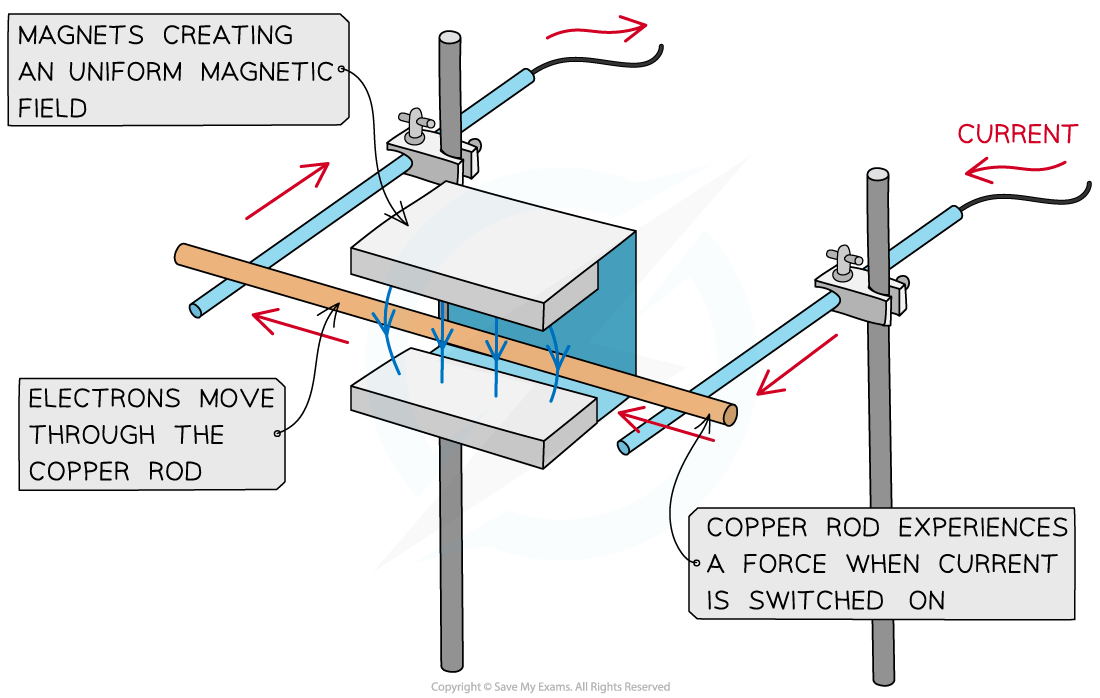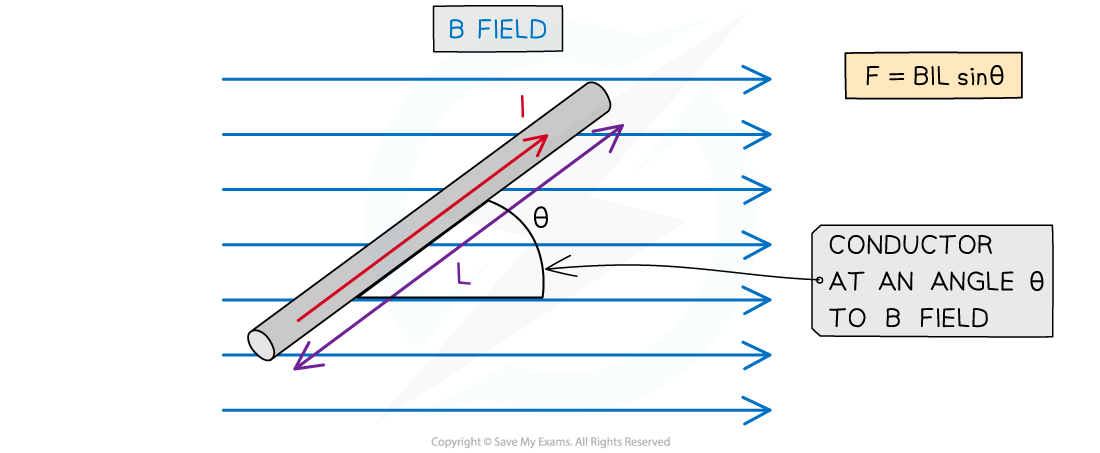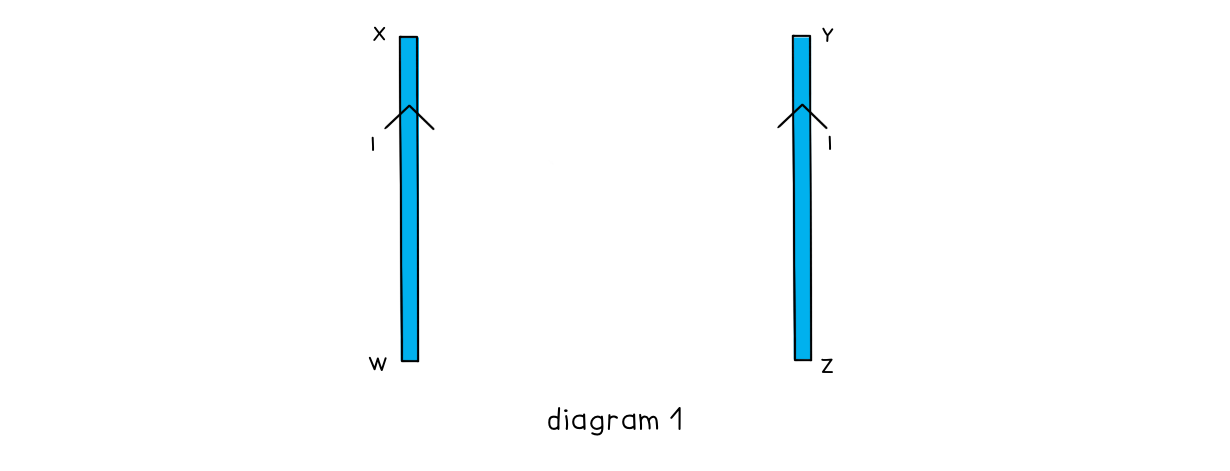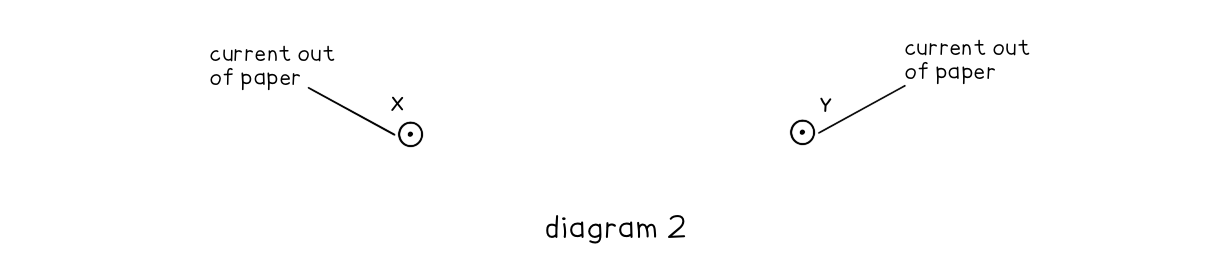# IB DP Physics: HL复习笔记5.4.3 Magnetic Force on a Current-Carrying Conductor

### Magnetic Force on a Current-Carrying Conductor

• A current-carrying conductor produces its own magnetic field
• When interacting with an external magnetic field, it will experience a force
• A current-carrying conductor will only experience a force if the current through it is perpendicular to the direction of the magnetic field lines
• A simple situation would be a copper rod placed within a uniform magnetic field
• When current is passed through the copper rod, it experiences a force that makes it moveA copper rod moves within a magnetic field when current is passed through it

#### Calculating Magnetic Force on a Current-Carrying Conductor

• The strength of a magnetic field is known as the magnetic flux density, B
• This is also known as the magnetic field strength
• It is measured in units of Tesla (T)
• The force F on a conductor carrying current I at right angles to a magnetic field with flux density B is defined by the equation

F = BIL sinθ

• Where:
• F = force on a current carrying conductor in a B field (N)
• B = magnetic flux density of external B field (T)
• I = current in the conductor (A)
• L = length of the conductor (m)
• θ = angle between the conductor and external B field (degrees)
• This equation shows that the greater the current or the magnetic field strength, the greater the force on the conductorMagnitude of the force on a current carrying conductor depends on the angle of the conductor to the external B field

• The maximum force occurs when sin θ = 1
• This means θ = 90o and the conductor is perpendicular to the B field
• This equation for the magnetic force now becomes:

F = BIL

• The minimum force (0) is when sin θ = 0
• This means θ = 0and the conductor is parallel to the B field
• It is important to note that a current-carrying conductor will experience no force if the current in the conductor is parallel to the field

#### Worked Example

A current of 0.87 A flows in a wire of length 1.4 m placed at 30o to a magnetic field of flux density 80 mT.Calculate the force on the wire.

Step 1: Write down the known quantities

Magnetic flux density, B = 80 mT = 80 × 10-3 T

Current, I = 0.87 A

Length of wire, L = 1.4 m

Angle between the wire and the magnetic field, θ = 30o

Step 2: Write down the equation for force on a current-carrying conductor

F = BIL sinθ

Step 3: Substitute in values and calculate

F =  (80 × 10-3) × (0.87) × (1.4) × sin(30) = 0.04872 = 0.049 N (2 s.f)

#### Origin of the Forces Between Current-Carrying Conductors

• A current carrying conductor, such as a wire, produces a magnetic field around it
• The direction of the field depends on the direction of the current through the wire
• This is determined by the right hand thumb rule
• Parallel current-carrying conductors will therefore either attract or repel each other
• If the currents are in the same direction in both conductors, the magnetic field lines between the conductors cancel out – the conductors will attract each other
• If the currents are in the opposite direction in both conductors, the magnetic field lines between the conductors push each other apart – the conductors will repel each otherBoth wires will attract if their currents are in the same direction and repel if in opposite directions

• When the conductors attract, the direction of the magnetic forces will be towards each other
• When the conductors repel, the direction of the magnetic forces will be away from each other
• The magnitude of each force depend on the amount of current and length of the wire

#### Worked Example

Two long, straight, current-carrying conductors, WX and YZ, are held at a close distance, as shown in diagram 1.The conductors each carry the same magnitude current in the same direction. A plan view from above the conductors is shown in diagram 2.On diagram 2, draw arrows, one in each case, to show the direction of:

• The magnetic field at X due to the current in wire YZ (label this arrow BYZ)
• The force at X as a result of the magnetic field due to the current in the wire YZ (label this arrow FYZ)
• The magnetic field at Y due to the current in wire WX (label this arrow BWX)
• The force at Y as a result of the magnetic field due to the current in the wire WX (label this arrow FWX)• Newton’s Third Law states:
• When two bodies interact, the force on one body is equal but opposite in direction to the force on the other body
• Therefore, the forces on the wires act in equal but opposite directions

#### Exam Tip

Remember that the direction of current flow is the flow of positive charge (positive to negative), and this is in the opposite direction to the flow of electrons